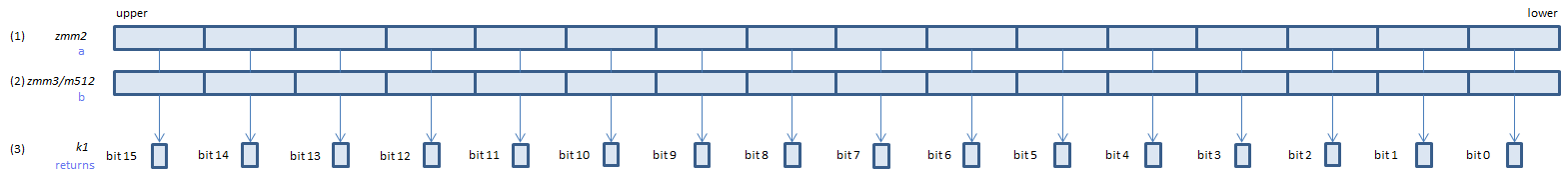﻿ vcmptrueps

## VCMPTRUEPS - CoMPare TRUE Packed Single

VCMPTRUEPS xmm1, xmm2, xmm3/m128    (V1
__m128 _mm_cmp_ps(__m128 a, __m128 b, _CMP_TRUE_UQ)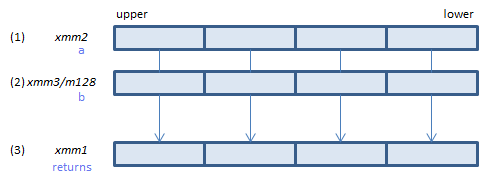For each float, always set 1 to all bits of the corresponding float of (3).
VCMPTRUEPS ymm1, ymm2, ymm3/m256    (V1
__m256 _mm256_cmp_ps(__m256 a, __m256 b, _CMP_TRUE_UQ)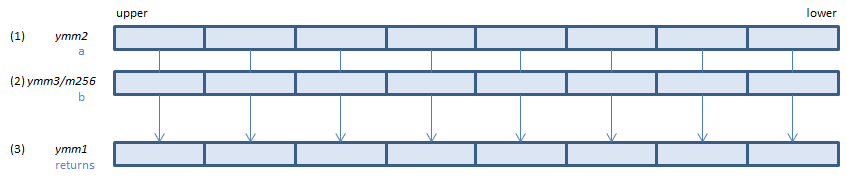For each float, always set 1 to all bits of the corresponding float of (3).
VCMPTRUEPS k1{k2}, xmm2, xmm3/m128/m32bcst    (V5+VL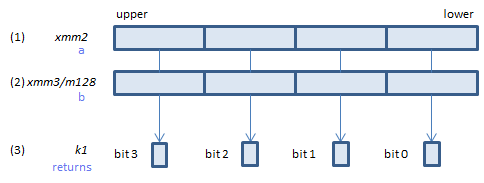For each float, always set 1 to the corresponding bit of (3).
If k2 bit is 0, the comparison is not done and the corresponding bit of (3) is set to zero. Upper bits of (3) are zero cleared.
VCMPTRUEPS k1{k2}, ymm2, ymm3/m256/m32bcst    (V5+VL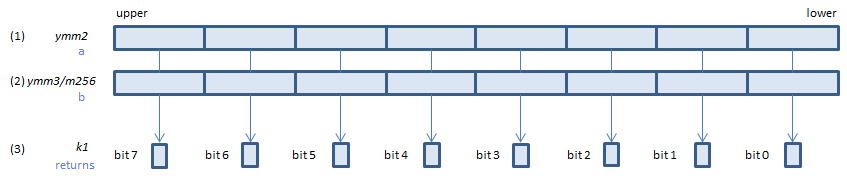For each float, always set 1 to the corresponding bit of (3).
If k2 bit is 0, the comparison is not done and the corresponding bit of (3) is set to zero. Upper bits of (3) are zero cleared.
VCMPTRUEPS k1{k2}, zmm2, zmm3/m512/m32bcst{sae}    (V5